Courses
Courses for Kids
Free study material
Free LIVE classes
More

# Rupees and Paise Class 3 Notes CBSE Maths Chapter 14 (Free PDF Download)LIVE
Join Vedantu’s FREE Mastercalss

## Solve Problems of Rupees and Paise Easily with Class 3 Maths Chapter 14 Revision Notes

Class 3 is the right stage to introduce the concept of money. Students will learn about rupees and paise in Chapter 14. These new concepts will become easier to understand by referring to Rupees and Paise revision notes. These notes have been composed by the top subject experts to benefit your study.

These notes will explain all the concepts related to money and will offer worksheets to practice. You will learn to identify the units of money prevalent in India and will enjoy doing the activities in this chapter.

Also, check CBSE Class 3 Maths revision notes for other chapters:

Last updated date: 21st Sep 2023
Total views: 142.5k
Views today: 3.42k

## Revision Notes Class 3 Mathematics Chapter 14 - Rupees and Paise

### Rupees

• The name Rupee has been derived from the Sanskrit word rupya.

• Rupee is the monetary unit from the 16th century.

• Countries like Mauritius, Nepal, and Seychelles also use the same monetary unit of Rupee.

• The silver Rupee was coined by the rupees Mughal Dynasty in the late 16th century and it was divided into 16 annas.

### Paise

• Paisa was introduced after the conversion of the system of currency of the Indian Rupee in 1957.

• Paisa is defined as the one-hundredth subdivision of Rupee.

• It exists as a monetary unit in several countries like Nepal, Pakistan etc.

### Solved Examples

1. How much money is represented by a given figure below?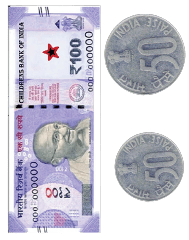Indian currency

Ans: The given money in the figure is One Hundred One Rupees total.

2. Use the notes and coins to show the following amounts of money

• Twenty-six Rupees

• Two hundred one Rupees

Ans2. To show Twenty-six Rupees we would need a 20 Rupee Note, a 5 Rupee Note and two 50 paise coins. Given figure below shows the required notes and coins.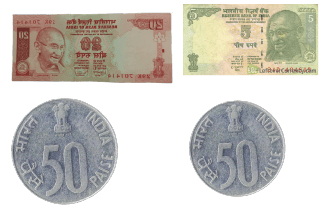Notes and Coins

To show Two hundred one Rupees we would need a 200 Rupee Note and a 1 Rupee coin.

Given figure below shows the required note and coin.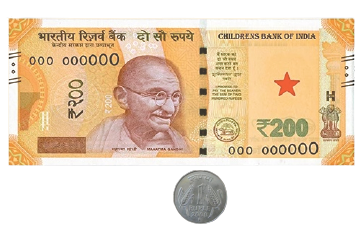A two hundred note and a coin

• In addition to the money we will learn how to add the amounts of money involving rupees and paise together.

• We carry out with money the same way as in decimal numbers.

•  While adding we need to follow that the amount of money is converted into paisa.

• Two different methods to solve addition involving rupees and paise. Students can practice both methods.

• (i) Adding the amounts with conversion into paisa

• (ii) Adding the amounts without conversion into paisa

• For example Rs25.30 + Rs40.43### Subtraction of money

• Subtraction in money by using the conversion method, we convert rupees and paise into paise and then subtract the smaller amount of paise from the greater amount.

• The obtained numbers are subtracted as ordinary numbers and if required finally we put a dot after two digits from the right.

• For example Rs72.98 - Rs32.84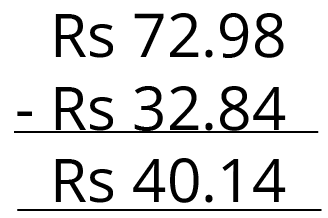Subtraction of money

### Solved Examples

1. The shirt cost Rs300 and a pair of shoes cost Rs700. Rohan buys a shirt and a pair of shoes. How much money does Rohan pay for the shirt and shoes together?

Ans1. Cost of shirt = Rs300

Cost of pair of shoes = Rs700

Total cost of the shirt and a pair of shoes = Cost of shirt + cost of pair of shoes

= Rs300 + Rs700

= Rs1000

Hence, Rohan would pay an amount of Rs1000 to buy a shirt and a pair of shoes together.

2. Akshay has total money of Rs2000. He buys a chair for Rs 1200 and a table for Rs 700. How much money is left with him?

Ans2. Total Money with Akshay = Rs2000

Cost of chair = Rs1200

Cost of Table = Rs700

Total money spent by Akshay = cost of Chair + cost of Table

= Rs1200 + Rs700

= Rs1900

Money left with Akshay = Rs2000 - R1900

= Rs100

### Practice Problems

Q1. Three friends Rohan, Ramesh, and Sumit wish to buy a cricket bat and ball. Rohan has Rs 48.50, Ramesh has Rs 55.50 and Sumit has Rs 38.00. Find the total money they have?

Q2. How much money is represented by a given figure below?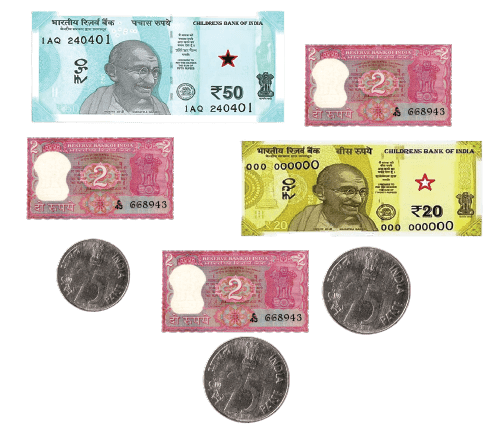How much is the given figure?

Q3. Sakshi and her friends went shopping. She purchased items for Rs 58, Rs 37 and Rs 22. Sakshi had a hundred-rupee note. How much money does she require to borrow from her friends to pay the bill?

Q4. This train goes from New Delhi to Mumbai. On its way, it stops at Jaipur, Ahmedabad and Gujarat stations.

Railway ticket cost to different stations has been given below in the table

 Distance from New Delhi(in km) STATION FARE (in Rs) 57 Jaipur 12.50 175 Ahmedabad 28.00 366 Gujarat 49.50 495 Mumbai 62.50

(i) Find the distance:

a) From Jaipur to Mumbai

b) Between Jaipur and Gujarat

e) From Gujarat to Mumbai

(ii) Find the cost of tickets:

a) Rohan is going from New Delhi to Ahmedabad. What is the cost of his ticket?

b) Sumit has to go from New Delhi to Gujarat. How much does he pay for the Ticket?

(c)Arun, Praveen and Shubham are going from New Delhi to Jaipur. What amount will be paid by them for three tickets? A 50 note is given for the tickets. How much money will they get back?

Ans1.  Rs 142

Ans2. 76 rupees 75 paise

Ans3. Rs 17

Ans4.

(i) (a) 438 km

b) 309 km

c) 320 km

d) 118 km

e) 129 km

(ii) (a) Rs 28

(b) Rs 49.50

(c) Distance between New Delhi to Jaipur = 57 km

The amount they pay for tickets = Rs 37.5

The money they received back = Rs 12.50

## Importance of Rupees and Paise Class 3 Chapter 14

This chapter comprises various activities and exercises that explain the different concepts of money to the Class 3 students. They will learn to differentiate between rupees and paise through easy examples. They will also learn to use these concepts to solve mathematical problems and answer questions.

The importance of this chapter can be summarised in the following points.

• Introduction to the concept of money and its prevalent units

• Various activities and exercises to understand the different forms of rupee notes and coins we use these days

• Understanding which coin or note is bigger or small in terms of value

• Playing money games and answering tricky questions to learn using money in real life

• Learning to calculate money by observing the pictures of notes and coins

• Calculating the value of items given in the exercise in terms of paisa money

• Using the concepts of addition, multiplication, and subtraction in calculating answers for the problem of rupees

## Benefits of Vedantu’s Money Chapter for Class 3 Revision Notes and Worksheets

• Quick comprehension of the concepts

By studying the Rupees and Paise summary given for all the sections, you can easily comprehend the concepts of money and learn how to calculate the value of items.

• Faster turnaround time

Resolve all your queries related to money rupees and make your preparation smoother. You will become capable of completing your preparation before an exam by using the revision notes as a reference.

• Identification and conversion of different monetary units

Use the simpler explanation of monetary units and learn how to convert 30 Paise in Rupees. Find out how to perform the addition of rupees and paise in different forms.

• Practising becomes easy

Find the money Chapter for Class 3 worksheet and solve the questions to practice.

Download the PDF file for free and learn how to convert rupees to paise worksheets. Develop your mathematical skills and understand the concept of money easily. Prepare for the exams by using these revision notes as references.

## FAQs on Rupees and Paise Class 3 Notes CBSE Maths Chapter 14 (Free PDF Download)

1. How can I calculate 1 rupee is equal to how many paise?

Focus on the units mentioned after the digits. Find out how to convert rupee to paisa and follow the methods of experts explained in the revision notes. You will learn how to convert rupees into paisa.

2. Is it necessary to learn what 1 paisa means?

The formation of 1 rupee occurs when you add 1 paisa 100 times. It means you must understand what ‘1 paise is equal to’ for finding the value of bigger coins and notes.